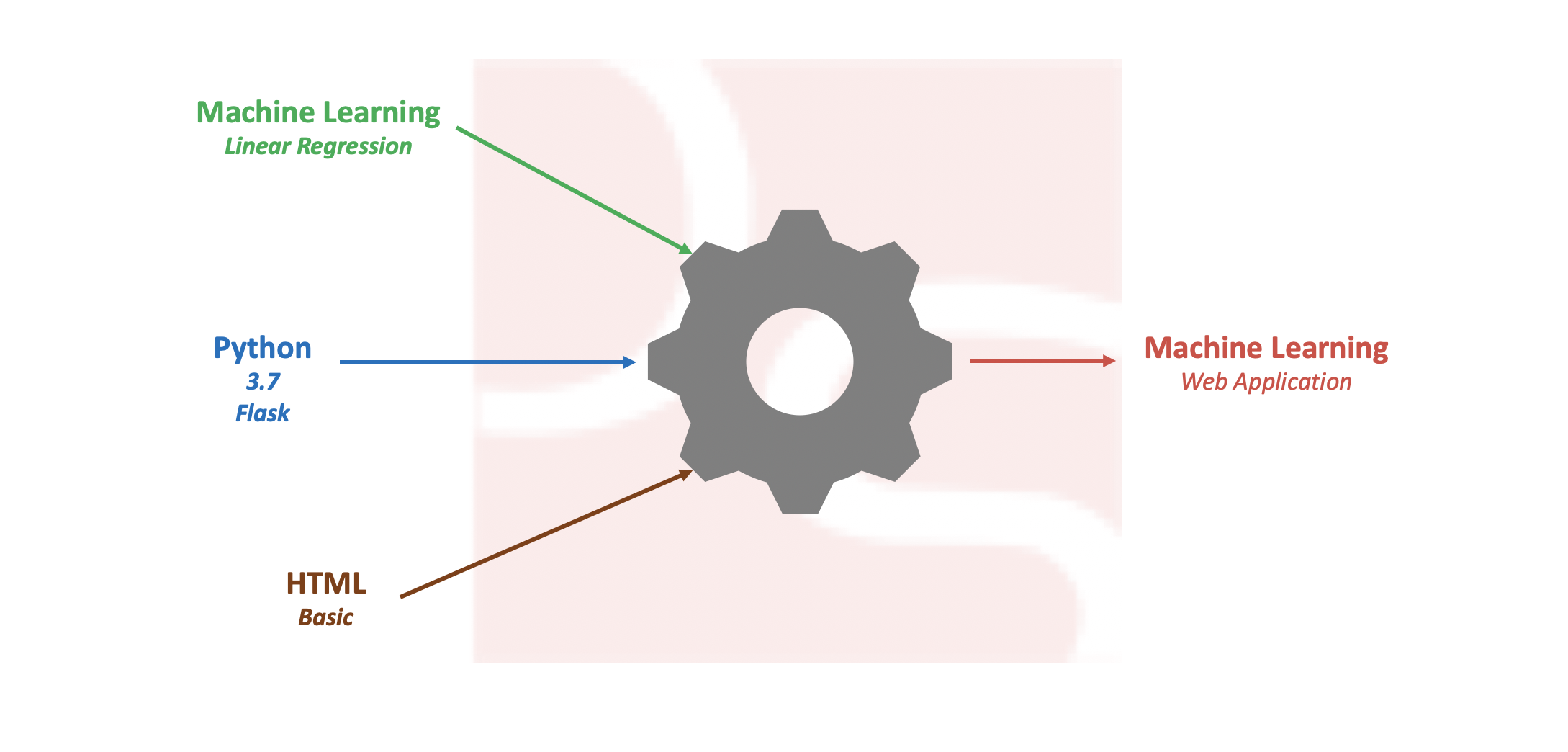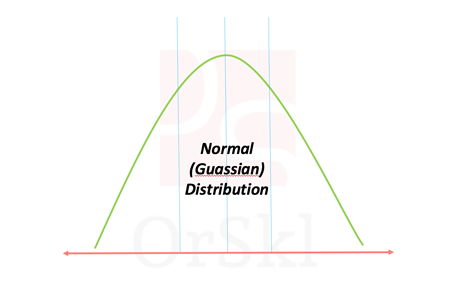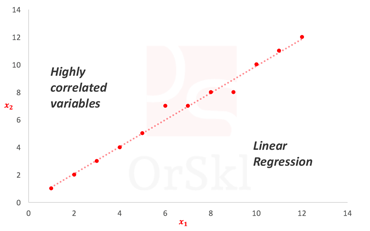##### Build Simple Machine Learning Web Application using Python

Pre-processing data and developing efficient model on a given data set is one of the daily tasks of machine learning engineer with commonly used languages like Python or R. Not every machine learning engineer would get a chance or requirement to integrate the model into real time applications like web or mobile for end users […]##### Ways to identify if data is Normally Distributed

Normal distribution also known as Gaussian distribution is one of the core probabilistic models to data scientists. Naturally occurring high volume data is approximated to follow normal distribution. According to Central limit theorem, large volume of data with multiple independent variables also assumed to follow normal distribution irrespective of their individual distributions. In reality we […]##### Will highly correlated variables impact Linear Regression?

Linear regression is one of the basic and widely used machine learning algorithms in the area of data science and analytics for predictions. Data scientists will deal with huge dimensions of data and it will be quite challenging to build simplest linear model possible. In this process of building the model there are possibilities of […]